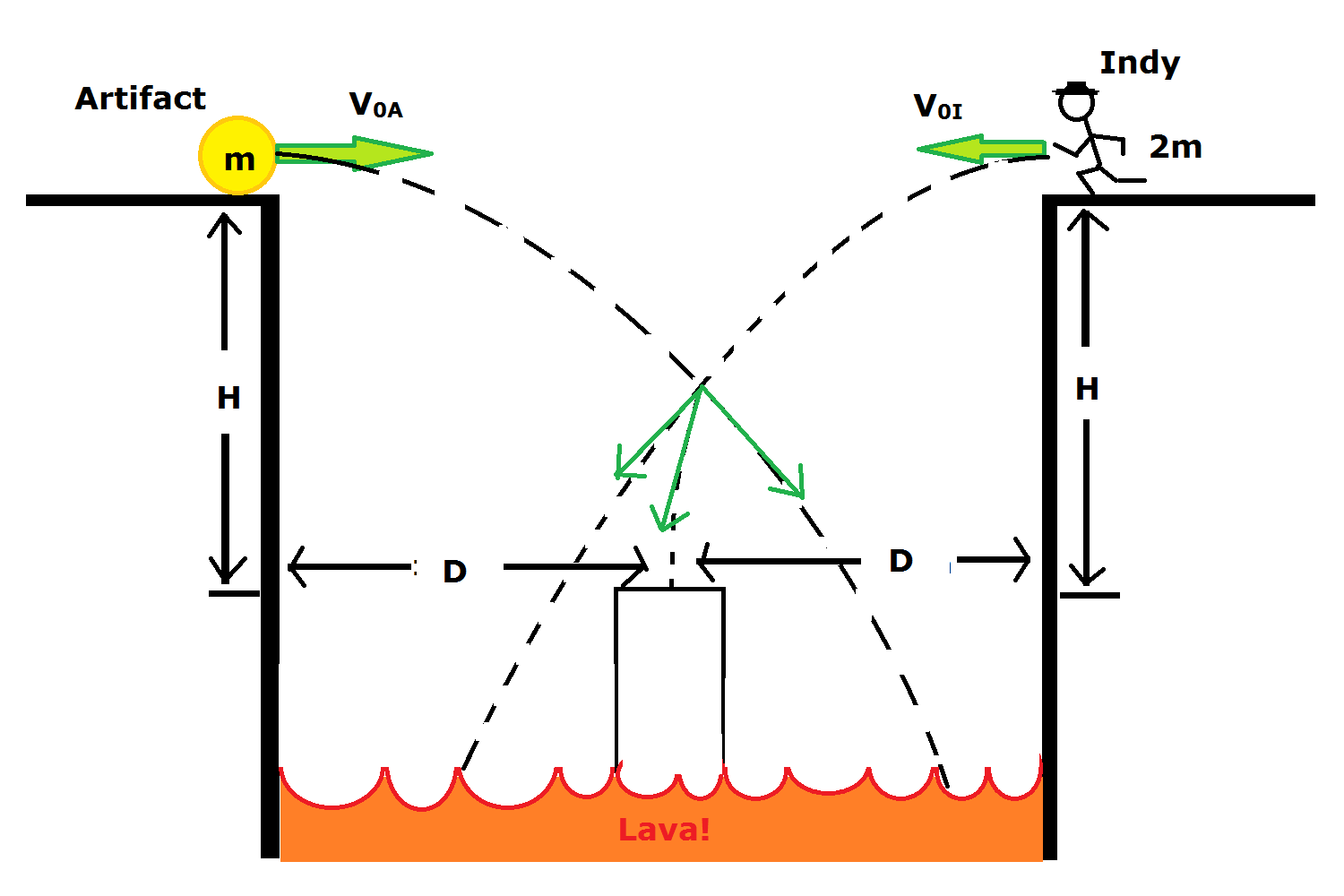# The Midair GrabAn ancient artifact is thrown horizontally off of a ledge overlooking a pool of lava. It has an initial speed of $v_{0A}$ and has mass $m$. There is a raised platform in the middle of the lava pool, at a distance $D$ to the left of the ledge, and $H$ below the ledge. The artifact is going to overshoot the platform.

Indiana Jones is standing on a ledge opposite the first ledge, at the same distance from the platform and the same elevation as the first ledge. He has mass $2m$. He takes a running horizontal leap off his ledge at the same instant the artifact is thrown.

If $v_{0A} = 20.2 \frac{m}{s}$, $D = 15.3 m$, and $H = 10.1 m$, how fast [$v_{0I}$] must Indy leap, so that he grabs the artifact and lands safely on the platform? Give your answer in $\frac{m}{s}$, rounded to 3 significant figures.

For acceleration due to gravity, use $g = 9.81 \frac{m}{s^{2}}$.

×